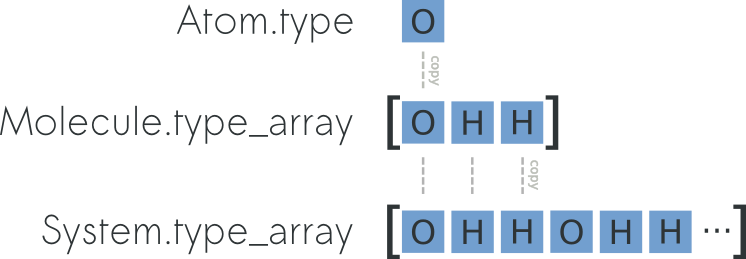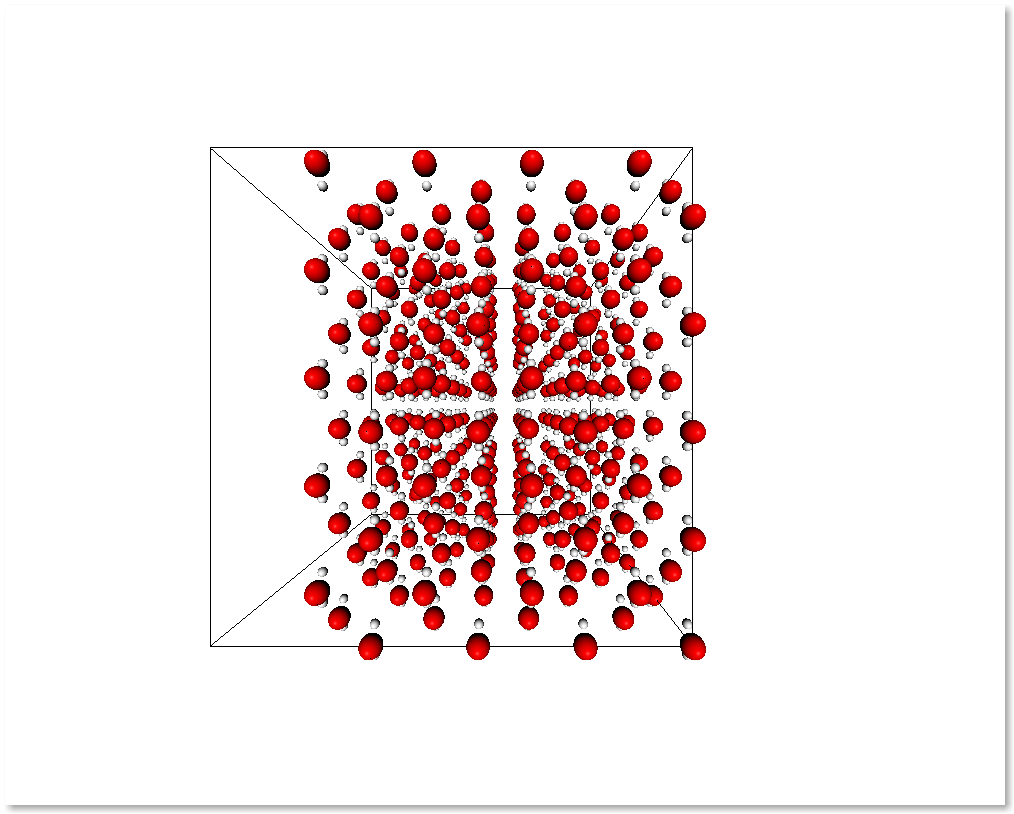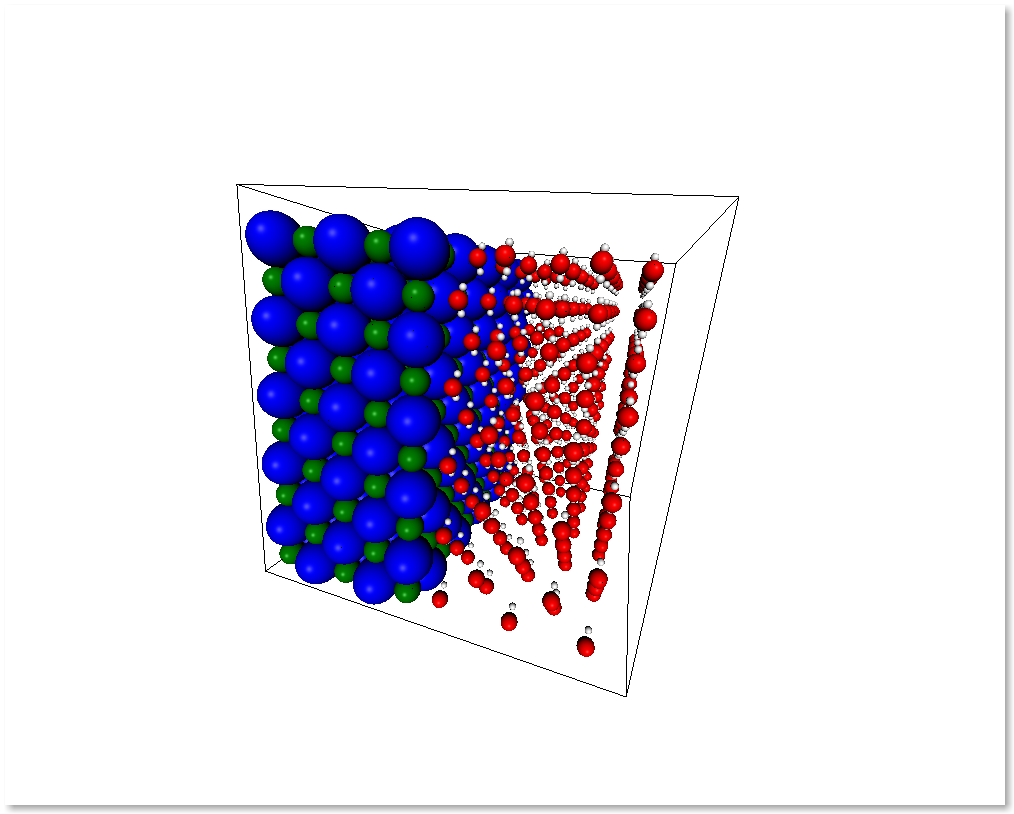# Atoms, Molecules and Systems¶

In chemlab, atoms can be represented using the chemlab.core.Atom data structure that contains some common information about our particles like type, mass and position. Atom instances are easily created by initializing them with data

>>> from chemlab.core import Atom
>>> ar = Atom('Ar', [0.0, 0.0, 0.0])
>>> ar.type
'Ar'
>>> ar.r
np.array([0.0, 0.0, 0.0])


Note

for the atomic coordinates you should use nanometers

A chemlab.core.Molecule is an entity composed of more atoms and most of the Molecule properties are inherited from the constituent atoms. To initialize a Molecule you can, for example, pass a list of atom instances to its constructor:

>>> from chemlab.core import Molecule
>>> mol = Molecule([at1, at2, at3])


## Manipulating Molecules¶

Molecules are easily and efficiently manipulated through the use of numpy arrays. One of the most useful arrays contained in Molecule is the array of coordinates Molecule.r_array. The array of coordinates is a numpy array of shape (NA,3) where NA is the number of atoms in the molecule. According to the numpy broadcasting rules, if you sum two arrays with shapes (NA,3) and (3,), each row of the first array gets summed with the second array. Let’s say we have a water molecule and we want to displace it randomly in a box, this is easily accomplished by initializing a Molecule at the origin and summing its coordinates with a random displacement:

import numpy as np

wat = Molecule([Atom("H", [0.0, 0.0, 0.0]),
Atom("H", [0.0, 1.0, 0.0]),
Atom("O", [0.0, 0.0, 1.0])], bonds=[[2, 0], [2, 1]])

# Shapes (NA, 3) and (3,)
wat.r_array += np.random.rand(3)


Using the same principles, you can also apply other kinds of transformations such as matrices. You can for example rotate the molecule by 90 degrees around the z-axis:

from chemlab.graphics.transformations import rotation_matrix

# The transformation module returns 4x4 matrices
M = rotation_matrix(np.pi/2, np.array([0.0, 0.0, 1.0]))[:3,:3]

for i,r in enumerate(wat.r_array):
wat.r_array[i] = np.dot(M,r)

# numpy efficient way to do the same:
# wat.r_array = np.dot(wat.r_array, M.T)


The array-based API provides a massive increase in performance and a more straightforward integration with C libraries thanks to the numpy arrays. This feature comes at a cost: the data is copied between atoms and molecules, in other words the changes in the costituents atoms are not reflected in the Molecule and vice-versa. Even if it may look a bit unnatural, this approach limits side effects and makes the code more predictable and easy to follow.

Bonds between atoms can be set or retrieved by using the bonds attribute. It’s an array of integers of dimensions (nbonds, 2) where the integer value corresponds to the atomic indices:

>>> from chemlab.db import ChemlabDB
>>> water = ChemlabDB().get('molecule', 'example.water')
>>> water.bonds
array([[0, 1],
[0, 2]])


By using the numpy.take function it’s very easy to extract properties relative to the bonds. numpy.take lets you index an array using another array as a source of indices, for example, we can extract the bonds extrema in this way:

>>> import numpy as np
>>> np.take(water.type_array, n.bonds)
array([['O', 'H'],
['O', 'H']], dtype=object)


If the array is not flat (like r_array), you can also specify the indexing axis; the following snippet can be used to retrieve the bond distances:

# With water.bonds[:, 0] we take an array with the indices of the
# first element of the bond. And we use numpy.take to use this array
# to index r_array. We index along the axis 0, along this axis
# the elements are 3D vectors.
>>> bond_starts = np.take(water.r_array, water.bonds[:, 0], axis=0)
>>> bond_ends = np.take(water.r_array, water.bonds[:, 1], axis=0)
>>> bond_vectors = bond_ends - bond_starts

# We sum the squares along the axis 1, this is equivalent of doint
# x**2 + y**2 + z**2 for each row of the bond_vectors array
>>> distances = np.sqrt((bond_vectors**2).sum(axis=1))
>>> print(distances)
[ 0.1         0.09999803]


Sometimes you don’t want to manually input the bonds, but want to have them automatically generated. In this case you may use the chemlab.core.Molecule.guess_bonds() method.

## Systems¶

In context such as molecular simulations it is customary to introduce a new data structure called System. A System represents a collection of molecules, and optionally (but recommended) you can pass also periodic box information:

>>> from chemlab.core import System
# molecule = a list of Molecule instances
>>> s = System(molecules, boxsize=2.0)


A System does not directly take Atom instances as its constituents, therefore if you need to simulate a system made of single atoms (say, a box of liquid Ar) you need to wrap the atoms into a Molecule:

>>> ar = Atom('Ar', [0.0, 0.0, 0.0])
>>> mol = Molecule([ar])


System, similarly to Molecule, can expose data by using arrays and it inherits atomic data from the constituent molecules. For instance, you can easily and efficiently access all the atomic coordinates by using the attribute System.r_array. To understand the relation between Atom.r, Molecule.r_array and System.r_array you can refer to the picture below:You can preallocate a System by using the classmethod System.empty (pretty much like you can preallocate numpy arrays with np.empty or np.zeros) and then add the molecules one by one:

import numpy as np
from chemlab.core import Atom, Molecule, System
from chemlab.graphics import display_system

# Template molecule
wat = Molecule([Atom('O', [0.00, 0.00, 0.01]),
Atom('H', [0.00, 0.08,-0.05]),
Atom('H', [0.00,-0.08,-0.05])])

# Initialize a system with four water molecules.
s = System.empty(4, 12) # 4 molecules, 12 atoms

for i in range(4):
wat.move_to(np.random.rand(3)) # randomly displace the water molecule
s.add(wat) # data gets copied each time

display_system(s)


Since the data is copied, the wat molecule acts as a template so you can move it around and keep adding it to the System.

Preallocating and adding molecules is a pretty fast way to build a System, but the fastest way (in terms of processing time) is to build the system by passing ready-made arrays, this is done by using chemlab.core.System.from_arrays().

Most of the chemlab.core.Molecule array attributes are still present in chemlab.core.System, including System.bonds; bonds between molecules are currently not supported and setting them will result in an unexpected behaviour. There is also a chemlab.core.System.guess_bonds() method to automatically set the intramolecular bonds.

### Building Systems¶

#### Random Boxes¶

It is possible to build boxes where atoms are placed randomly by using the chemlab.core.random_lattice_box() function. A set of template molecules are copied and translated randomly on the points of a 3d lattice. This ensures that the spacing between molecules is consistent and to avoid overlaps.

To make an example box:

from chemlab.db import ChemlabDB
from chemlab.core import random_lattice_box

# Example water molecule
water = ChemlabDB().get('molecule', 'example.water')

s = random_lattice_box([water], , [4.0, 4.0, 4.0])


#### Crystals¶

chemlab provides an handy way to build crystal structures from the atomic coordinates and the space group information. If you have the crystallographic data, you can easily build a crystal:

from chemlab.core import Atom, Molecule, crystal
from chemlab.graphics import display_system

# Molecule templates
na = Molecule([Atom('Na', [0.0, 0.0, 0.0])])
cl = Molecule([Atom('Cl', [0.0, 0.0, 0.0])])

s = crystal([[0.0, 0.0, 0.0], [0.5, 0.5, 0.5]], # Fractional Positions
[na, cl], # Molecules
225, # Space Group
cellpar = [.54, .54, .54, 90, 90, 90], # unit cell parameters
repetitions = [5, 5, 5]) # unit cell repetitions in each direction

display_system(s)


Note

If you’d like to implement a .cif file reader, you’re welcome! Drop a patch on github.

### Manipulating Systems¶

#### Selections¶

You can manipulate systems by using some simple but flexible functions. It is really easy to generate a system by selecting a part from a bigger system, this is implemented in the functions chemlab.core.subsystem_from_atoms() and chemlab.core.subsystem_from_molecules().

Those two functions take as the first argument the original System, and as the second argument a selection. A selection is either a boolean array that is True when we want to select that element and False otherwise, or an integer array containing the elements that we want to select. By using those two functions we can create a subsystem by building those selections.

The following example shows an easy way to take the molecules that contain atoms in the region of space x > 0.5 by employing subsystem_from_atoms():

import numpy as np
from chemlab.core import crystal, Molecule, Atom, subsystem_from_atoms
from chemlab.graphics import display_system

# Template molecule
wat = Molecule([Atom('O', [0.00, 0.00, 0.01]),
Atom('H', [0.00, 0.08,-0.05]),
Atom('H', [0.00,-0.08,-0.05])])

s = crystal([[0.0, 0.0, 0.0]], [wat], 225,
cellpar = [.54, .54, .54, 90, 90, 90], # unit cell parameters
repetitions = [5, 5, 5]) # unit cell repetitions in each direction

selection = s.r_array[:, 0] > 0.5
sub_s = subsystem_from_atoms(s, selection)

display_system(sub_s)It is also possible to select a subsystem by selecting specific molecules; in the following example we select the first 10 water molecules by using subsystem_from_molecules():

from chemlab.core import subsystem_from_molecules

selection = np.array([0, 1, 2, 3, 4, 5, 6, 7, 8, 9])
sub_s = subsystem_from_molecules(s, selection)


Note

chemlab will provide other selection utilities in the future, if you have a specific request, file an issue on github

#### Merging systems¶

You can also create a system by merging two different systems. In the following example we will see how to make a NaCl/H2O interface by using chemlab.core.merge_systems():

import numpy as np
from chemlab.core import Atom, Molecule, crystal
from chemlab.core import subsystem_from_atoms, merge_systems
from chemlab.graphics import display_system

# Make water crystal
wat = Molecule([Atom('O', [0.00, 0.00, 0.01]),
Atom('H', [0.00, 0.08,-0.05]),
Atom('H', [0.00,-0.08,-0.05])])

water_crystal = crystal([[0.0, 0.0, 0.0]], [wat], 225,
cellpar = [.54, .54, .54, 90, 90, 90], # unit cell parameters
repetitions = [5, 5, 5]) # unit cell repetitions in each direction

# Make nacl crystal
na = Molecule([Atom('Na', [0.0, 0.0, 0.0])])
cl = Molecule([Atom('Cl', [0.0, 0.0, 0.0])])

nacl_crystal = crystal([[0.0, 0.0, 0.0], [0.5, 0.5, 0.5]], [na, cl], 225,
cellpar = [.54, .54, .54, 90, 90, 90],
repetitions = [5, 5, 5])

water_half = subsystem_from_atoms(water_crystal,
water_crystal.r_array[:,0] > 1.2)
nacl_half = subsystem_from_atoms(nacl_crystal,
nacl_crystal.r_array[:,0] < 1.2)

interface = merge_systems(water_half, nacl_half)
display_system(interface)At the present time, the merging will avoid overlapping by creating a bounding box around the two systems and removing the molecules of the first system that are inside the second system bounding box. In the future there will be more clever ways to handle this overlaps.

#### Removing¶

There are two methods used to remove specific atoms and molecules from a system. chemlab.core.System.remove_molecules() and chemlab.core.System.remove_atoms(). Taking from the previous NaCl example, you may need to remove some excess ions to meet the electroneutrality condition:

# n_na and n_cl are the number of Na and Cl molecules
toremove = 'Na' if n_na > n_cl else 'Cl'
nremove = abs(n_na - n_cl) # Number of indices to be removed

remove_indices = (s.type_array == toremove).nonzero()[:nremove]

s.remove_atoms(rem_indices)


#### Sorting and reordering¶

It is possible to reorder the molecules in a System by using the method chemlab.core.System.reorder_molecules() that takes the new order as the first argument. Reordering can be useful for example to sort the molecules against a certain key.

If you use chemlab in conjunction with GROMACS, you may use the chemlab.core.System.sort() to sort the molecules according to their molecular formulas before exporting. The topology file expect to have a file with the same molecule type ordererd.

## Extending the base types¶

Warning

This part of chemlab is still in draft. This first, very brief implementation serves as a specification document. As we collect more feedback and feature requests there will be an expansion and a refinement of the extension functionalities.

Differents applications of chemistry may require additional data attached to each atom, molecule or system. For example you may need the velocity of the system, atomic charges or number of electrons. Chemlab should be able to provide a way to simply attach this data while retaining the selection and sorting functionalities.

The management of the atomic and molecular properties within a System is done through specific handlers. Those handlers are called attributes and fields. In the following example we may see how it’s possible to add a new field “v” to the Atom class, and transmit this field as a “v_array” in the Molecule and System class. In those cases they basically take as their argument the attribute/field name, the type, and a function that return the default value for the field/attribute:

from chemlab.core.attributes import MArrayAttr, NDArrayAttr
from chemlab.core.fields import AtomicField

class MyAtom(Atom):
fields = Atom.fields + [AtomicField("v",
default=lambda at: np.zeros(3, np.float))]

class MyMolecule(Molecule):
attributes = Molecule.attributes + [MArrayAttr("v_array", "v", np.float,
default=lambda mol: np.zeros((mol.n_atoms, 3), np.float))]

class MySystem(System):
attributes = System.attributes + [NDArrayAttr("v_array", "v_array", np.float, 3)]


Those class are ready to use. You may want to create new instances with the Atom.from_fields, Molecule.from_arrays and System.from_arrays.

Once you’ve done your field-specific job with MyAtom/MyMolecule/MySystem you can convert back to a chemlab default class class by using the astype methods:

at = myat.astype(Atom)
mol = mymol.astype(Molecule)
sys = mysys.astype(System)